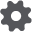# Linear Spring

The damped Linear Spring contact force model is based on the work by Cundall and Strack (Cundall and Strack 1979). A linear spring with stiffness k is in parallel with a dashpot with coefficient, c. The magnitude of the normal force between two particles, FN, is:where k is the linear spring stiffness, c is the dashpot coefficient, δ is the overlap, and δdot is the overlap velocity. A similar force can be applied to the tangential direction.

The spring stiffness and the dashpot coefficient are the parameters in this model and it is a common practice to estimate the spring stiffness and calculate the dashpot coefficient based on this stiffness. The simulation time step is then estimated based on the spring stiffness.

The spring constant and dashpot coefficient can be calculated based on a combination of material properties and kinematic constraints. One common method is obtained by equating the maximum strain energy in a purely Hertzian contact (Ehertzian) with the maximum strain energy of the existing contact (Emax). This gives:where the equivalent mass m*, the equivalent radius R* and the equivalent Young’s modulus E* were defined earlier. V is the typical impact velocity.

For two identical spherical particles with masses of 7.63e-03 kg, radius of 9 mm and Young’s modulus of 2.6e+08 Pa, colliding at a velocity of 3 m/s, k ≈ 2.0e+05 N/m.

The impact velocity in an EDEM simulation can usually be taken as a characteristic velocity in the simulation. You can base this velocity as the maximum velocity in the simulation, for example, for a blending operation with the blender operating at Ω rad/s, the characteristic velocity is equal to r Ω m/s, where r is the radius of the blender. The dashpot coefficient is related to the coefficient of restitution as:where e is the coefficient of restitution. Note that e remains constant with the impact speed (assuming other model parameters are constant).

The tangential stiffness is usually estimated as a ratio to the normal spring stiffness (Cundall and Strack 1979). EDEM has the tangential stiffness equal to the normal stiffness.

The dashpot coefficient is calculated using the tangential stiffness in the equation above. The tangential force is computed via:where kt and ct are the tangential spring and dashpot coefficient, μ is the coefficient of friction.

The simulation time step is usually a small percentage of the contact duration of the particles. The contact duration for the Linear Spring model is obtained using the normal stiffness as:where β = π /ln(e) and e is the coefficient of restitution.

For e = 0.5 we have a contact time of 0.00043 sec. The simulation time step must be less than this value for better integration of the particle states. We recommend you have a value of about 5-10 % of this contact time for accurate results. The details of the soft particle contact model are relatively unimportant due to the fact that a lumped parameter approach which neglects the details of the contact force (e.g. a coefficient of restitution) is sufficient to describe the collision dynamics.

Note that you can increase the simulation time step and then try to fix a stiffness that will not allow for excessive overlap. However, since the stiffness and time step are not based on physical laws, the accuracy of the results is not guaranteed: you might obtain a qualitative similarity but not a quantitative one. We recommend to calculate the stiffness based on the material properties and fix the time step in EDEM.

There is no general consensus on what the best contact model is. The Linear Spring model is simpler than Hertz-Mindlin due to less computational overhead. However, in both models the contact force is discontinuous at the first and last point of contact, and energy dissipation is poor in systems with small relative velocities.

For the same stiffness, we obtain a larger force for the same time step in a Hertz-Mindlin model in comparison with the Linear Spring model. Hence a larger time step can be used with a Linear Spring contact model.

Remove the default Hertz-Mindlin (no-slip) contact model from the list when using this model.

 Interaction Configurable Parameters Position Particle to Particle, Particle to Geometry Set the characteristic velocity of the spring for the material interaction. Be sure the velocity is the same for both particle-to-particle and particle-to-geometry. Last

Use:

1. Choose Edit Contact Chain > Base Model and select Linear Spring. Choose any rolling friction or additional models as required.
2. Click the configuration buttonto define contact model parameters.